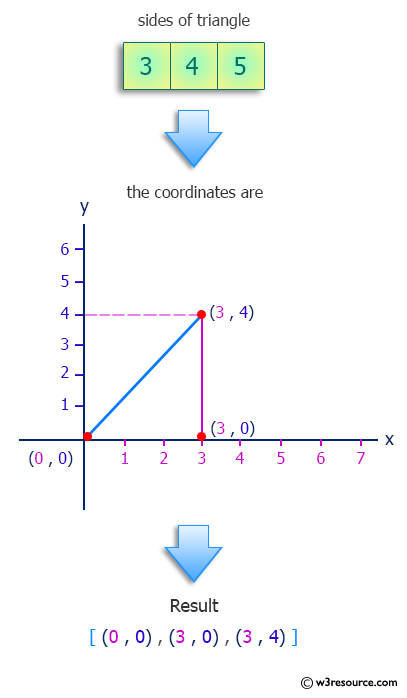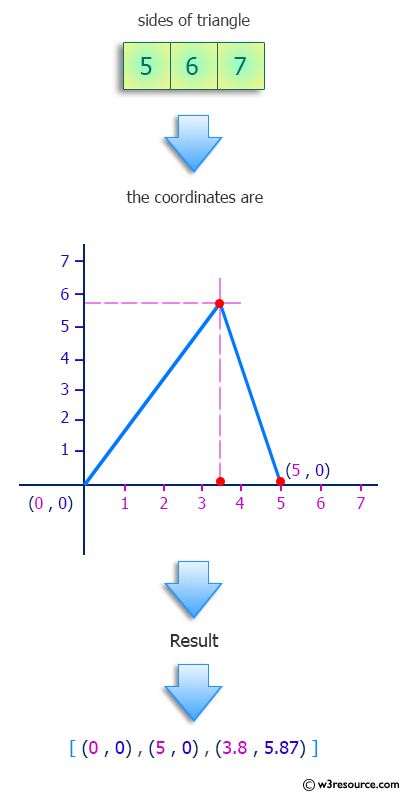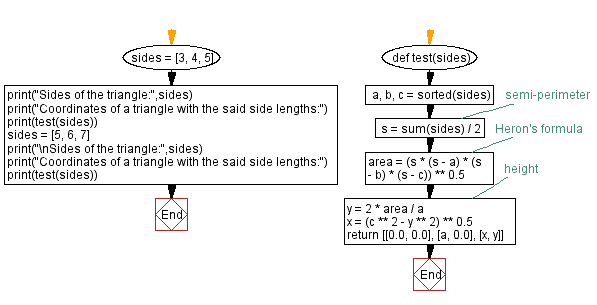﻿ Python: Find the coordinates of a triangle with the given side lengths - w3resource# Python: Find the coordinates of a triangle with the given side lengths

## Python Programming Puzzles: Exercise-31 with Solution

Write a Python program to find the coordinates of a triangle with the given side lengths.

```Input:
[3, 4, 5]
Output:
[[0.0, 0.0], [3, 0.0], [3.0, 4.0]]

Input:
[5, 6, 7]
Output:
[[0.0, 0.0], [5, 0.0], [3.8, 5.878775382679628]]
```

Pictorial Presentation:Sample Solution:

Python Code:

``````#License: https://bit.ly/3oLErEI

def test(sides):
a, b, c = sorted(sides)
s = sum(sides) / 2 # semi-perimeter
area = (s * (s - a) * (s - b) * (s - c)) ** 0.5 # Heron's formula
y = 2 * area / a # height
x = (c ** 2 - y ** 2) ** 0.5
return [[0.0, 0.0], [a, 0.0], [x, y]]
sides = [3, 4, 5]
print("Sides of the triangle:",sides)
print("Coordinates of a triangle with the said side lengths:")
print(test(sides))
sides = [5, 6, 7]
print("\nSides of the triangle:",sides)
print("Coordinates of a triangle with the said side lengths:")
print(test(sides))
``````

Sample Output:

```Sides of the triangle: [3, 4, 5]
Coordinates of a triangle with the said side lengths:
[[0.0, 0.0], [3, 0.0], [3.0, 4.0]]

Sides of the triangle: [5, 6, 7]
Coordinates of a triangle with the said side lengths:
[[0.0, 0.0], [5, 0.0], [3.8, 5.878775382679628]]
```

Flowchart:## Visualize Python code execution:

The following tool visualize what the computer is doing step-by-step as it executes the said program:

Python Code Editor :

Have another way to solve this solution? Contribute your code (and comments) through Disqus.

What is the difficulty level of this exercise?

Test your Programming skills with w3resource's quiz.

﻿

## Python: Tips of the Day

Clamps num within the inclusive range specified by the boundary values x and y:

Example:

```def tips_clamp_num(num,x,y):
return max(min(num, max(x, y)), min(x, y))
print(tips_clamp_num(2, 4, 6))
print(tips_clamp_num(1, -1, -6))
```

Output:

```4
-1
```